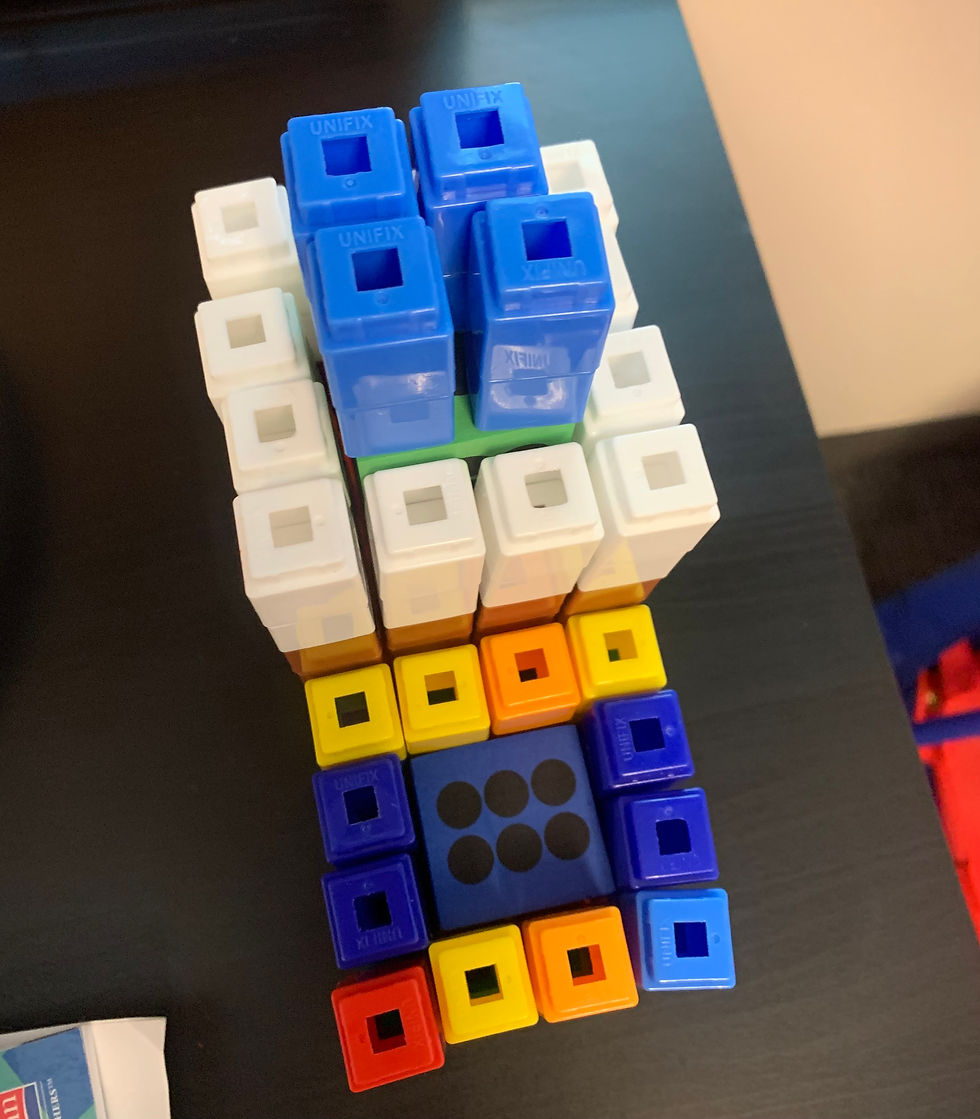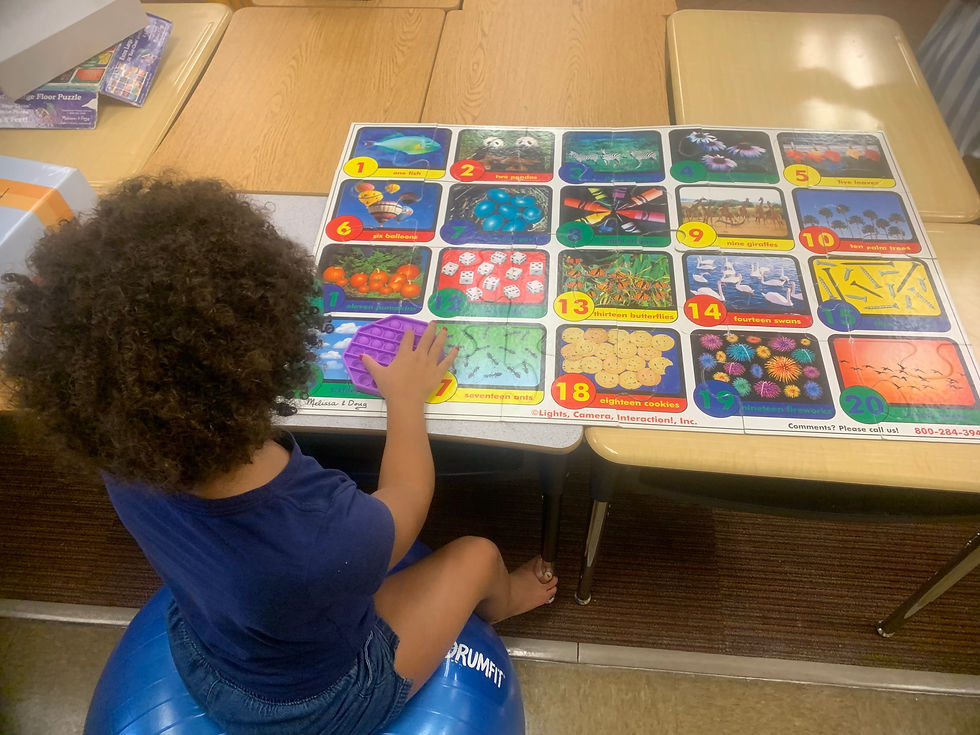top of page
Search
• drlynnekenney

# 5 Steps to Building Conceptual Thinking Skills in Numeracy

Updated: Feb 3

Teaching fundamental math skills is central to long-term enjoyment and success in math. Students with dyslexia, dyscalculia, and dyspraxia often have underlying deficits in the connections in the areas of the brain that allow for rapid, fluid, and comprehensive numeracy understanding. Combining patterned coordinative rhythmic movement with foundational numeracy concepts is how we "Play" rather than "Teach" math.Building cognitive readiness, an understanding of mathematical relationships, and the ability to implement "the language of math" are dependent on developing some of the following numeracy skills.

“Numeracy is the ability to execute standard arithmetic operations

(addition, subtraction, multiplication, and division) on whole numbers

correctly, consistently, and fluently with understanding. The numerate child

is able to estimate, calculate accurately and efficiently, both mentally and on

paper using a range of calculation strategies and means”, Sharma, 2013.

1. Number Relationships - This is the first skill we discover in Play Math.

We begin with simple games where we roll dice or play cards and “build” the numbers we see with Unifix blocks. Our students do well when they can “see” that a number “looks like”. Using kinesthetic strategies to discover and manipulate number relationships builds creativity and confidence for our students.· Using narrative language with visual observation skills we introduce single digit addition, and single digit subtraction with numbers from 0-10; then 10-24. You can do a lot of number play with numbers from 0-24. You can build 2’s 4’s 6’s 8’s 10’s and 12’s all making up 24. You can then divide those “towers of blocks” showing what happens when we make “sets” of numbers from 0-24.

· We then move to seeing how “numbers fit into other numbers” by building multiplication and division arrays with Unifix blocks, cubes, and drawing.

· We teach the concept, “how many” of “what kinds” of numbers do we see?

· 3 x 5 is 3 (how many) of 5’s (what kind)

· 5 x 3 is 5 (how many) of 3’s (what kind)

This is a fun discovery process as children as young as five years of age begin to “see” the commutative property and observe that multiplication is simply fast addition. In multiplication we are making copies of sets of things (fish, erasers, blocks, cupcakes etc).

2. Pattern Recognition - Pattern Recognition for students with dyscalculia is a core skill. Being able to see, make, describe and manipulate patterns underlies how they engage mathematically with confidence. Here we play subitizing, addition, subtraction, multiplication, division and exponential activities.

Pattern Recognition is the ability to see and recognize patterns, a skill that is necessary for encoding and understanding fact families, times tables, multiplication and division facts, exponential growth, and equations. A central feature of pattern play is to explore, discover and experience how numbers fit together and are related to one another.

READ the 2020 IES Practice Guide on Elementary Math

3. Series and Sequences - We introduce the concept that quantities represented by numbers can be viewed as “series and sequences”. We begin by drawing a number line and wondering aloud, what numbers will we find when we “hop” across the number line in 2’s? How about when we hop in 3’s?

A mathematical series is the sum of a list of numbers that are generating according to some pattern or rule (1 + 3 + 5 + 7).

A sequence is an ordered list of elements. In math, it’s usually numbers (2, 4, 6, 8) yet it can also be objects (cupcakes, lollipops, animals).Sequencing is a skill needed for working with number lines, math charts, place value, multi-step math processing, counting forward and backward, and following the order of operations.

4. Factors! Series, sequencing, and pattern activities lead us to discover factors. A factor is a number that fits evenly into another number. As an example, 3 fits into 12, 4 times. Therefore, 3 and 4 are factors of 12.

· Every number will have a minimum of two factors, 1 and the number itself.

· A number that has only two factors, 1 and the number itself, is called a prime number. A prime number is only divisible by 1 and itself.

At this point in Play Math have experienced building, making, creating, and manipulating numerical relationships that make sense. Moving onto skills such as visualization we bring in a good deal of “mental math”. We help the students examine the patterns when moving up or down the number line in sets (groups) of numbers leading to many “Ah-Ha!” “I see it” moments. Creating the mental picture and "seeing" numerical relationships is key.

5. Our fifth foundational concept in Play Math is Magic 10’s and 5’s Alive. Most of our students come to us having heard how to count by 10’s and 5’s. Using these quantities as anchors we can do multistep equations by asking ourselves, “What do I need to get to 5 or 10”? These activities build mathematical thinking skills.

There are other concepts besides composition and decomposition that build a mathematical thinker. Yet we observe in clinical practice that when students are introduced to the base ten system in a way that they can think-through the composing and decomposing of numbers, it begins to make sense and they actually like "being detectives" and "cognitive scientists in order to think-through math.

It is a joy working with a student with language and numeracy challenges in a manner that they can now “see” “understand” and “explain” number relationships.

Incorporating pattern recognition, sequencing, subitizing, visualization, mental manipulation, estimation, and the concrete building of numbers makes all the difference for these students. It’s an honor to bring math to life with confidence and creative exploration with students who experience challenges in numeracy.

Developmental-Conceptual Math Books We Recommend:

Lisa Zack-Swasey, M.Ed., SAIF Assessing Mathematics Pre-requisite Skills.

Mahesh C. Sharma, (2003). Factors Responsible for Mathematics Learning. Center for Teaching/Learning of Mathematics: Cambridge, MA.

Thomas P. Carpenter (2016). Young Children’s Mathematics: Cognitively Guided Instruction in Early Childhood Education.

Liping Ma (1999). Knowing and Teaching Elementary Mathematics.

Stanislas Dehaene (2011). The Number Sense: How the Mind Creates Mathematics, Revised and Updated Edition